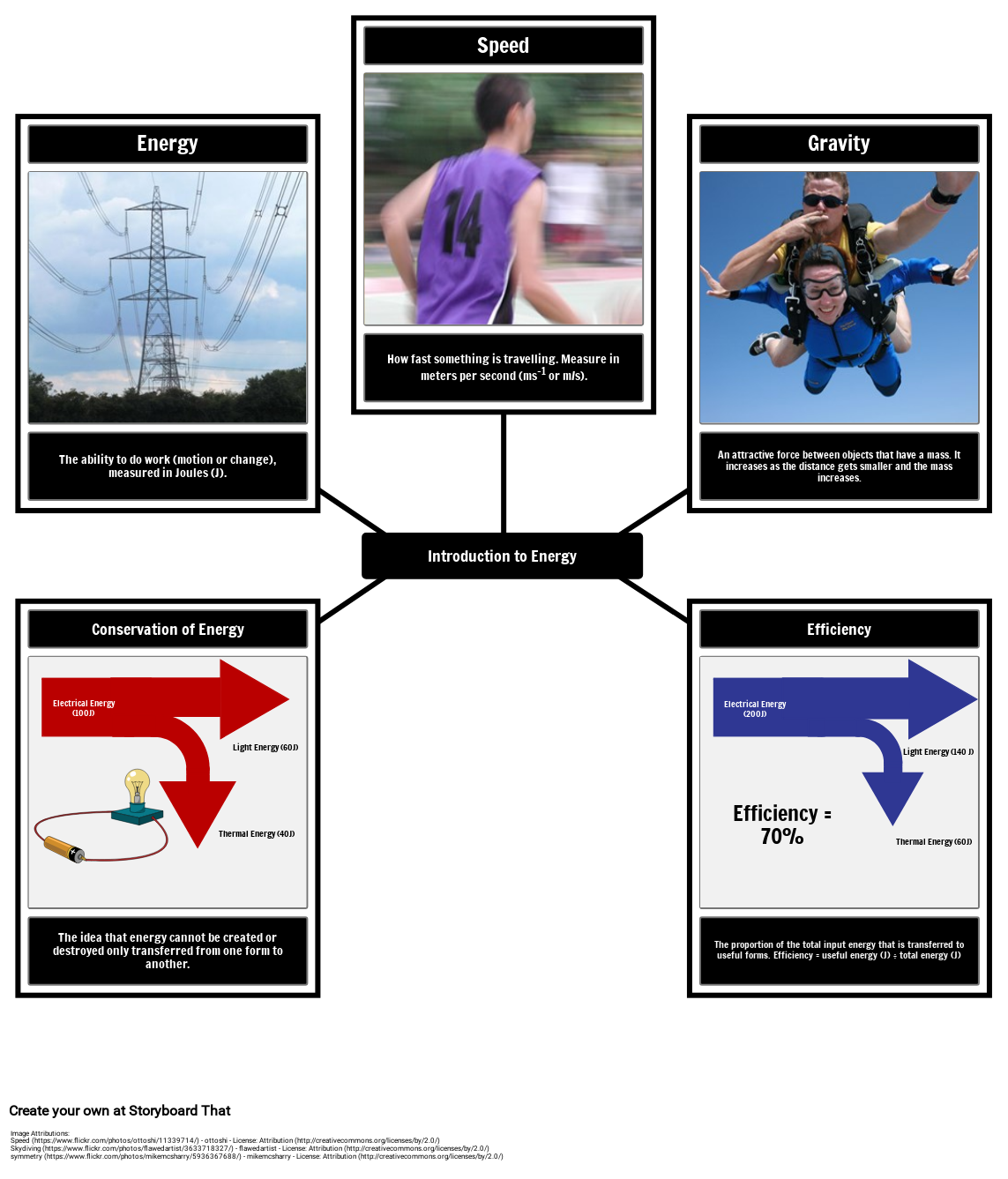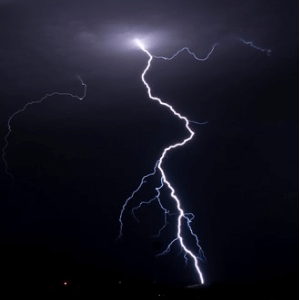# Introduction to Energy VocabularyYou can find this storyboard in the following articles and resources:### Introduction to Energy

By Oliver Smith

Energy cannot be created or destroyed, and this concept is known as the conservation of energy. Because of this, the universe has a finite amount of energy which is transferred from one form to another. In the early 20th century, Einstein put forth the idea that energy and mass were interchangeable, leading to the most famous equation in Science: E=mc2. The activities in this lesson plan explore different forms of energy and energy transfers through the creation of visual aids.

### Introduction to Energy

#### Storyboard Description

What is Energy? Energy Vocabulary Example

#### Storyboard Text

• Energy
• Speed
• Gravity
• The ability to do work (motion or change), measured in Joules (J).
• How fast something is travelling. Measure in meters per second (ms-1 or m/s).
• An attractive force between objects that have a mass. It increases as the distance gets smaller and the mass increases.
• Conservation of Energy
• Electrical Energy (100J)﻿
• Light Energy (60J)﻿
• Introduction to Energy
• Efficiency
• Electrical Energy (200J)﻿
• ﻿Efficiency = 70%
• Light Energy (140 J)﻿
• The idea that energy cannot be created or destroyed only transferred from one form to another.
• Thermal Energy (40J)﻿
• The proportion of the total input energy that is transferred to useful forms. Efficiency = useful energy (J) ÷ total energy (J)
• Thermal Energy (60J)﻿